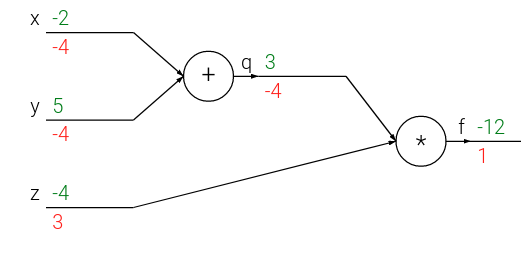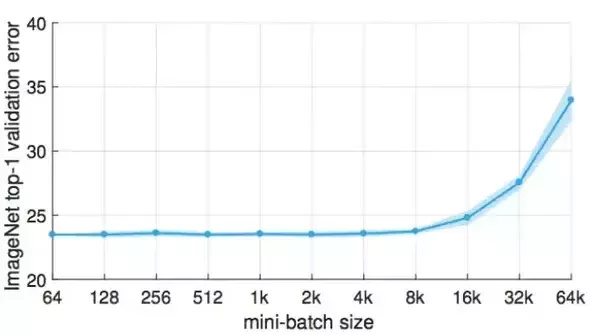It is a basic question, but I haven’t fully grasped it yet.

1- SGD vs. GD
Why don’t we use GD in practice? And would it be slower if we do GD and then instead of small step (lr) like SGD, we change the weights by a large step (suppose we don’t have memory/computation issue)

2- I understand how backprop works in this simple example:But I don’t know how to generalize it when we have a batch of two (for example). My guess is we do it two times for each sample in batch and then average the gradient (Is it right?)

PS: I copied the picture from http://cs231n.github.io/optimization-2/

@jeremy Your explanation is always the bestWith regards to combining the loss for each example, the answer lies in the cost function. It is what ties the output on each training example together to produce a single number, the loss on the batch. It is this single number that we backpropagate from and how the loss function combines contributions on a per example basis is what will dictate what we need to do when looking at the gradients.

The cost function that we have used the most thus far in the course is (I believe) categorical cross entropy. It calculates the loss for each example and takes the average.

With regards to why SGD and not GD even if we were operating without memory constraints, I am not sure what the answer to this is. Personally, I suspect the randomness of updates has some nice properties where if you find yourself at a saddle point slightly different loss across batches might make it easier for you to get out of it, but whether this is indeed the case, I do not know.

In practical terms, there is also the notion that the examples you train on will share some similarities. The updates on reasonable batch size should not be that much different from what you would get on the entire training set and taking smaller but more frequent steps in roughly correct direction will allow you to find a good solution faster than taking bigger steps but less frequently in the ideal direction. There is also the consideration that with gradient updates we do not know what is ideal in the absolute sense of the word - we only figure out where to move based on the information available to us locally (the slope of the function). This might be another reason why more steps that let us explore more of the weight space faster might be a better ideaOn the other hand, there was a paper published recently I believe (and referenced on the forums somewhere) that if you make your learning rate sufficiently big, training with large batch sizes will be equivalent to training with smaller batch sizes and you will require less parameter updates (which is nice if you want to distribute the computation).

For larger datasets large batchsizes are not feasible but I am fairly sure you could stick an entire MNIST on the GPU (maybe even CIFAR10?) so potentially one could experiment with this further even on a single GPU3 Likes

I don’t think this is the article that you were referring to, but has some interesting results for larger batch sizes anyway3 Likes

This would help.

This video exactly tells you batch vs mini-batch.

Actually I don’t think the mini-batch size needs to be 1 for SGD.
SGD is characterized by not using all the training data to compute the gradients, but instead a portion of it, so I think any mini-batch size that is smaller than the number of training samples will be considered SGD. But yeah, generally we use a very small mini-batch size compared to the number of training samples.

3 Likes

Going back to the memory issue, i think the answer might lie in number of computations that you do for GD which takes the whole dataset for step while SGD takes some n number of examples to calcualte the next step to take. When you iterate over a large dataset, i think its proven that SGD does get very close to where GD might take you as well. But SGD seems very prone to local minima as well.